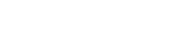# math.asin

`FLOATmath.asinFLOATx`

Available inall subroutines.

Computes the principal value of the arc sine of the argument `x`.

## Parameters

`x` - Floating point value. The value of `x` should be in the range -1 to 1 inclusive.

## Return Value

Upon successful completion, this function returns the arc sine of `x`, in the range -`math.PI_2` to `math.PI_2` radians inclusive.

If `x` is `math.NAN`, a NaN will be returned.

If `x` is ±0, `x` will be returned.

If `x` is `math.POS_INFINITY` or `math.NEG_INFINITY`, a domain error occurs and a NaN will be returned.

If `x` is subnormal, a range error occurs and `x` will be returned.

For finite values of x not in the range -1 to 1 inclusive, a domain error occurs and a NaN will be returned.

## Errors

• If the `x` argument is finite and is not in the range -1 to 1 inclusive, or is `math.POS_INFINITY` or `math.NEG_INFINITY`, then `fastly.error` will be set to `EDOM`.
• If the `x` argument is subnormal, then `fastly.error` will be set to `ERANGE`.

## Example

``````declare local var.fo FLOAT;
set var.fo = math.asin(1.0);``````

BETA